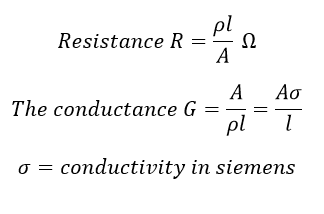# What is Conductance

## What is Conductance:

Conductance and Resistance are opponent phenomena. When a substance or material can conduct better, we say that its resistance is less. When it conducts less, we say that its resistance is high. But most of the time electrical engineers don’t mention resistance or a material rather they prefer to speak about conductance of a material.

The reciprocal of resistance is called conductance.Conductance is the ability of a substance or material to pass electricity. The measure of the opposite of resistance is conductance. Siemens, S is the unit of conductance. When the resistance of a component is 1 ohm then its conductance is also 1 Siemens. When the resistance is increased to double, the conductance is decreased to half and vice-versa.

The relation between Siemens and ohms can be written as:

Siemens = 1/ohms, or

ohms =1/Siemens

### Resistivity Vs Conductivity of The Material

Material ρ (Ω·m) at 20 °C σ (S/m) at 20 °C
Silver 1.59×10−8 6.30×107
Copper 1.68×10−8 5.96×107
Annealed copper 1.72×10−8 5.80×107
Gold 2.44×10−8 4.10×107
Aluminium 2.65×10−8 3.77×107
Calcium 3.36×10−8 2.98×107
Tungsten 5.60×10−8 1.79×107
Zinc 5.90×10−8 1.69×107
Nickel 6.99×10−8 1.43×107
Lithium 9.28×10−8 1.08×107
Iron 9.71×10−8 1.00×107
Platinum 1.06×10−7 9.43×106
Tin 1.09×10−7 9.17×106
Gallium 1.40×10−7 7.10×106
Carbon steel (1010) 1.43×10−7 6.99×106
Titanium 4.20×10−7 2.38×106
Grain oriented electrical steel 4.60×10−7 2.17×106
Manganin 4.82×10−7 2.07×106
Constantan 4.90×10−7 2.04×106
Stainless steel 6.90×10−7 1.45×106
Mercury 9.80×10−7 1.02×106
Nichrome 1.10×10−6 6.7×105
GaAs 1.00×10−3 to 1.00×108 1.00×10−8 to 103
Carbon (amorphous) 5.00×10−4 to 8.00×10−4 1.25×103 to 2×103
Carbon (graphite) 2.50×10−6 to 5.00×10−6 ∥basal plane 2.00×105 to 3.00×105 ∥basal plane
3.00×10−3 ⊥basal plane 3.30×102 ⊥basal plane
Germanium 4.60×10−1 2.17
Seawater 2.00×10−1 4.8
Swimming pool water 3.33×10−1 to 4.00×10−1 0.25 to 0.30
Drinking water 2.00×101 to 2.00×103 5.00×10−4 to 5.00×10−2
Silicon 6.40×102 1.56×10−3
Wood (damp) 1.00×103 to 1.00×104 10−4 to 10−3
Deionized water 1.80×105 5.50×10−6
Glass 1.00×1011 to 1.00×1015 10−15 to 10−11
Hard rubber 1.00×1013 10−14
Wood (oven dry) 1.00×1014 to 1.00×1016 10−16 to 10−14
Sulfur 1.00×1015 10−16
Air 1.30×1014 to 3.30×1014 3×10−15 to 8×10−15
Carbon (diamond) 1.00×1012 ~10−13
Fused quartz 7.50×1017 1.30×10−18
PET 1.00×1021 10−21
Teflon 1.00×1023 to 1.00×1025 10−25 to 10−23